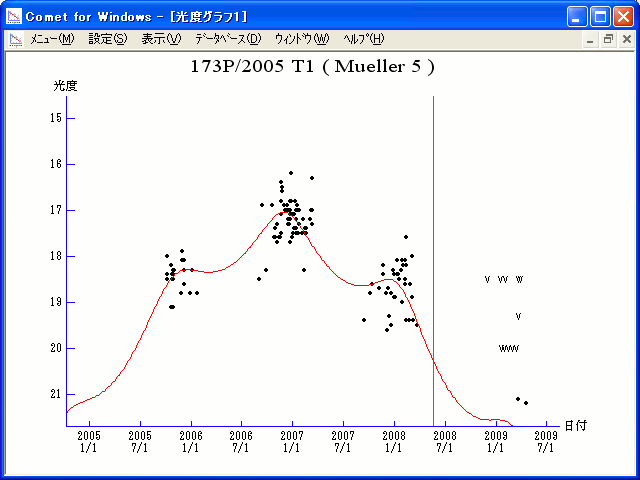# \$B%_%e%i!

173P/Mueller 5 (2008)###\$B%W%m%U%#!<%k(B

 \$BId9f(B 173P/2005 T1 \$B8!=PF|(B 2005\$BG/(B10\$B7n(B7\$BF|(B \$B8!=P8wEY(B 18.0\$BEy(B \$B8!=P E. J. Christensen (Mt. Lemmon Survey)

###\$B###\$B50F;MWAG(B

```                    Epoch = 2008 May  14.0 TT
T = 2008 May  18.5222 TT         Peri. =  29.8348
e = 0.261149                     Node  = 100.5697  2000.0
q = 4.214478 AU                  Incl. =  16.4959
a =  5.704097 AU    n = 0.0723475    P =  13.62 years
```

###\$B@1?^(B###\$B8wEYJQ2=(B

```        m1 = -14 + 5 log\$B&\$(B + 45 log r(t + 600)
```##### \$B50F;MWAG\$O(B Dr. Brian G. Marsden \$B\$N7W;;\$K\$h\$k\$b\$N\$G!"(B IAUC 8613 \$B\$K7G:\\$5\$l\$?\$b\$N\$G\$9!#(B \$B@1?^\$O(B StellaNavigator Ver.2.0 for Windows (\$B%"%9%H%m%"!<%D(B \$BJTCx(B / \$B%"%9%-!<=PHG6I4)(B) \$B\$G:n@.\$7\$?\$b\$N\$G\$9!#(B \$B8wEY%0%i%U\$O(BComet for Windows\$B\$G:n@.\$7\$?\$b\$N\$G\$9!#(B